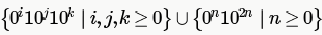Courses

# Context Free Language - 2

## 15 Questions MCQ Test RRB JE for Computer Science Engineering | Context Free Language - 2

Description
This mock test of Context Free Language - 2 for Computer Science Engineering (CSE) helps you for every Computer Science Engineering (CSE) entrance exam. This contains 15 Multiple Choice Questions for Computer Science Engineering (CSE) Context Free Language - 2 (mcq) to study with solutions a complete question bank. The solved questions answers in this Context Free Language - 2 quiz give you a good mix of easy questions and tough questions. Computer Science Engineering (CSE) students definitely take this Context Free Language - 2 exercise for a better result in the exam. You can find other Context Free Language - 2 extra questions, long questions & short questions for Computer Science Engineering (CSE) on EduRev as well by searching above.
QUESTION: 1

### Which of the following statements is true?

Solution:

(A) is wrong as a language can be context free even if it is being accepted by non deterministic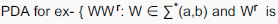reverse}

(C) and (D) not always true as Context free languages are not closed under Complement and Intersection.

QUESTION: 2

### Let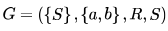be a context free grammar where the rule set R is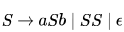Which of the following statements is true?

Solution:

It will be easy to analyze the problem if we replace terminal a and b by ( and ) respectively.

S →(S) | SS | ε

L(G) = balanced parentheses [each left parenthesis has a matching right parenthesis and are well nested ]

example () , ()(), (()), (()()()).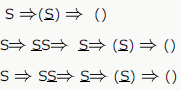String () can be derived by above three way each having different derivation tree.

So G is Ambiguous
b) Concatenation of two balance parenthesis will be balanced also . eq. x = (()) y= () xy= (())().
c) We can design Deterministic PDA for L. put left parenthesis (only) in stack and pop with right parenthesis.
d) We cannot design DFA for L because we need a stack to match left parenthesis with right parenthesis.
only option C is true.

QUESTION: 3

### Consider the languages: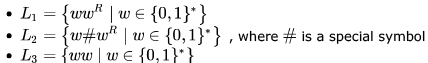Which one of the following is TRUE?

Solution:
QUESTION: 4

Let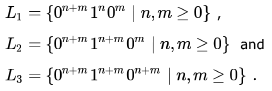Which of these languages are NOT context free?

Solution:

L1 is context-free. We count the number of 0's and check if the remaining number of 1's followed by 0's count to the initial
number of 0's.

L2 is not context-free. Here the number of 0's and the following 1's must be same, which can be checked using a PDA. But
after that we must also ensure that the following number of 0's must be less than the previous count of 0's and 1's
(otherwise n < 0, which violates the condition for acceptance) and we cannot do these two checks using a single PDA.

L3 is again not context-free as it is nothing but equal number of 0's followed by equal number of 1's followed by equal
number of 0's.

QUESTION: 5

In the context-free grammar below, S is the start symbol, a and b are terminals, and ϵ denotes the empty string.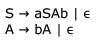The grammar generates the language

Solution: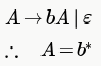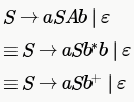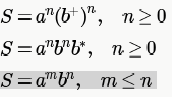QUESTION: 6

In the context-free grammar below, S is the start symbol, a and b are terminals, and ϵ denotes the empty string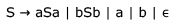Which of the following strings is NOT generated by the grammar?

Solution:

L(G) = PALINDROME
baba does not belong to palindrome , so B is the answer

QUESTION: 7

The two grammars given below generate a language over the alphabet {x, y, z}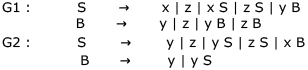Which one of the following choices describes the properties satisfied by the strings in these languages?

Solution:

from Above grammar
Regular expression for G1: (x+z)+ + (x+z)*y(y+z)+

Regular expression for G2 :(y+z+xy)+

QUESTION: 8

Consider the grammar given below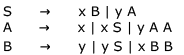Consider the following strings.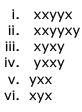Which of the above strings are generated by the grammar ?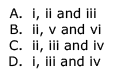Solution:

Above grammar is for equal no of x and y

from Non-terminal S -> xB

=>xy [as B->y one y for one x]

S->xB
=> xxBB [as B ->yBB one B result in one y for one x ]

S->xB
=>xyS [as B->yS one y for one x and start again]
Note :Same applies for string start with y i.e . S->yA

QUESTION: 9

Consider the following grammars. Names representing terminals have been specified in capital letters.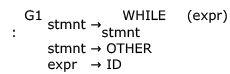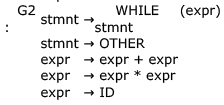Which one of the following statements is true?

Solution:

Regular grammar is either right linear or left linear. A left linear grammar is one in which there is at most 1 non-terminal on the right side of any production, and it appears at the left most position. Similarly, in right linear grammar non-terminal appears at the right most position.

Here, we can write a right linear grammar for G1 as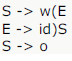(w - WHILE, o - OTHER)

So, L(G1) is regular.

Now for G2 also we can write a right linear grammar: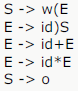making its language regular.

So, both G1 and G2 have an equivalent regular grammar. But as such both these grammars are neither right linear nor left
linear and hence not a regular grammar. So, D must be the answer.

QUESTION: 10

A CFG G is given with the following productions where S is the start symbol, A is a non-terminal and a and b are terminals.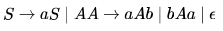Which of the following strings is generated by the grammar above?

Solution:

S→ aS
S→ aA
S→ aaAb
S→ aabAab
S→ aabbAaab
S→ aabbaab
hence d is d answer

QUESTION: 11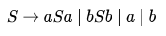The language generated by the above grammar over the alphabet  is the set of

Solution:

string generated by this language is a,b,aba,bab,aabaa,.....all this strings are odd length palindromes

QUESTION: 12

Which of the following languages are context-free?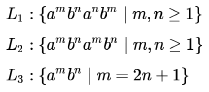Solution:

first check for L1. now look a^m&b^m and a^n&b^n must b comparable using one stack for CFL. now take a stack push all a^m in to the stack then push all b^n in to stack now a^n is coming so pop b^n for each a^n by this b^n and a^n will b comparable. now we have left only a^m in stack and b^m is coming so pop a^m for each b^m by which we can compare a^m to b^m ..we conclude that we are comparing this L1 using a single stack so this is CFG.

now for L2.this can not be done in to a single stack because m and n are not comparable we can not find when to push or pop so this is CSL.

now for L3.push all a's into stack and pop 2a 's for every b and at last we left with a single a .
bcz here aaaaabb is a valid string where m=2n+1 and n=2.So realized using single stack hence L3 is CFG.

QUESTION: 13

Consider the following context-free grammar over the alphabet ∑  = {a,b,c } with S as the start symbol: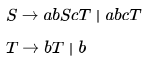Which one of the following represents the language generated by the above grammar?

Solution:

Consider the 1st production S-> abScT
This production generates equal number of (ab)'s and c's but after each c there is T which goes to T->bT

so with each c there can be one or more b's (one because of production T->b and more because of T->bT)

and these b's are independent.

For example

ababcbbcbb is the part of the langugage
and ababcbbbbbbcbb is also the part of the language so we rule out A and C both say equal number of b's after each c

In option D equal number of (ab)'s and c's is not satisfied . The only option that satisfies these 2 conditions is B

number of (ab)'s and c's is not satisfied . The only option that satisfies these 2 conditions is B

people who says this will be the string at any case

S-> abcT

-> abcb

no problem for i B in this case also here cmn=cm1 means n value 1 thats it

and remember in B c is not coming eternally.it is coming until m value reaches n of b..right there

.if m=n=1 then cb stops

QUESTION: 14

If G is a grammar with productions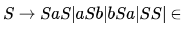where S is the start variable, then which one of the following strings is not generated by G ?

Solution:
QUESTION: 15

Consider a CFG with the following productions.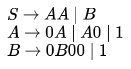S is the start symbol, A and B are non-terminals and 0 and 1 are the terminals. The language generated by this grammar is

Solution: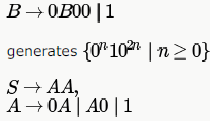generates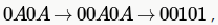which is there in only B and D choices. D is not the answer as "00" is not generated by the given grammar. So, only option left is B and if we see carefully, non-terminal B is generating the second part of B choice and AA is generating the first part.